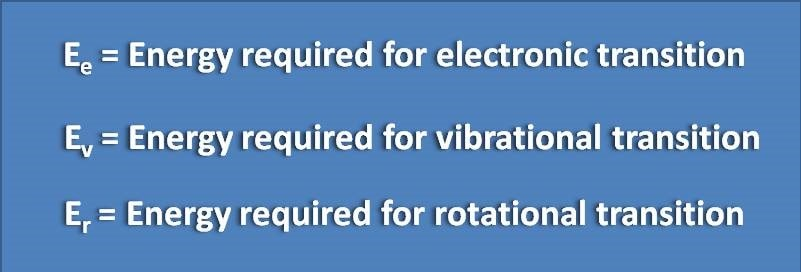1. Home
2. â€º
3. Test papers
4. â€º

# MCQ on UV-Visible spectroscopy: Page-7

1. Diffraction gratings work on the basis of

(A) Max-Well Boltzman’s equation

(B) Bragg’s equation

(C) Noise-whitney equation

(D) Beer’s law

When two parallel beam of lights incident at angle of θ on a diffraction surface, they produce an interference due to difference in their path lengths. This phenomenon is used in diffraction gratings which work by Bragg’s equation. This equation given as nλ=2d sinθ

2. Select INCORRECT statement related to the following data.(A) E e > E v > E r

(B) E e=100 * E v

(C) E e=10000 * E r

(D) E r=100* E v

When a electromagnetic radiation falls on analyte, it can undergo three types of transitions such as electronic, vibrational and rotational transitions. The energy required for these three transitions will be in the ratio of 10000 : 100 : 1 respectively.

3. Dark current in photo multiplier tube is

(A) The current produced by falling light on cathode

(B) The current produced by falling light on anode

(C) The residual current due to spontaneous discharge of dynodes

(D) The residual current that appears after the removal of incident light

Photomultiplier tube consists of number of electrodes called as dynodes in between cathode and anode. These dynodes amplify the signal so that even a small signal can be easily detected. Sometimes a small current may be produced from spontaneous discharge of these dynodes resulting in a small reading in detector even the analyte is not placed. This current is called as dark current.

4. The mid IR region is

(A) 400-800 nm

(B) 25 μm-2.5 μm

(C)4000-400 cm-1

(D) 2.5 μm – 1mm

Mid IR region comprises of 4000 to 400 cm -1 which is equal to 2.5 µm to 25 µm in terms of wavelength.

5. Choose the wave number corresponding to 25 μm

(A) 2500 cm -1

(B) 4000 cm-1

(C) 250 cm -1

(D) 400 cm -1

Wave number is the reciprocal of wavelength. ν=1/λ. Therefore we can convert 25 μm into wave number as follows.